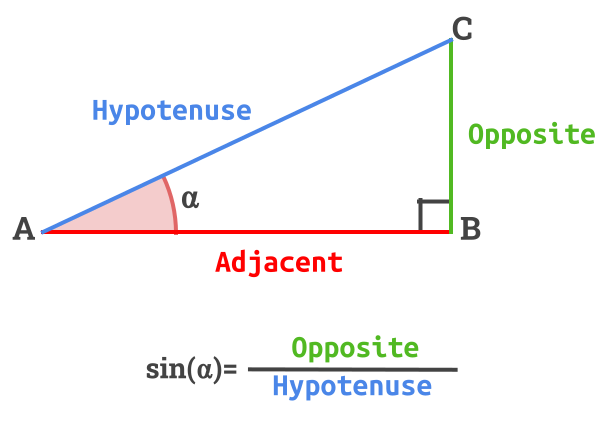👋 Hello! I'm Alphonsio the robot. Ask me a question, I'll try to answer.

# What is the sine equation in a right-angled triangle?

In the following right-angled triangle :The equation of the sine is given by :

﻿﻿

More